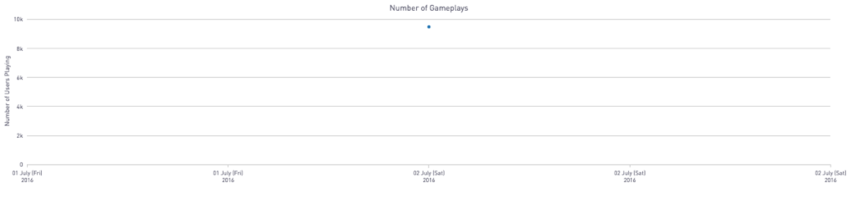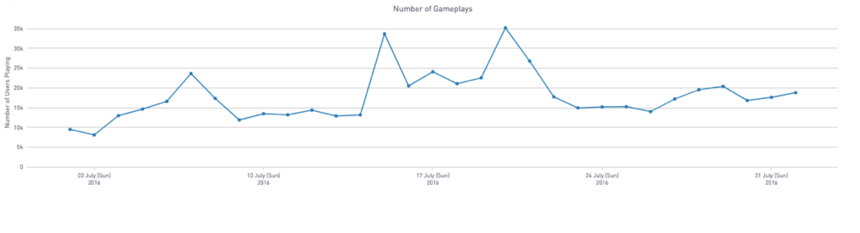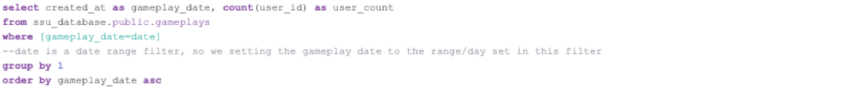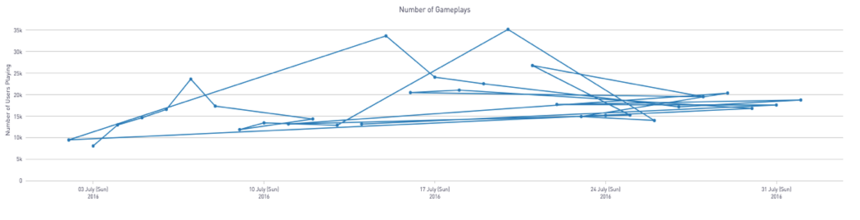# Dynamic Line or Scatter Chart Based on a Date Range Filter

Single Scatter Point ChartLine Chart for Multiple Data PointsBackground

Let’s say that we would like a dynamic chart where we can visualize the number of users who started a game session over the course of a month as a line chart, but also have the functionality that when a user chooses a single date/datapoint (in a filter), it is displayed as a single scatter point.

In some cases, depending on the filter applied in the SQL query, either a single data point or multiple data points can be returned. If the preference is to mix chart types depending on the resulting data point/s, then we can leverage our Plot.ly integration to generate a dynamic chart. For this analysis, we will be using our publicly available demo dataset which entails user gameplays by platform (i.e. web, android, iOS) and date.

SQL Query:Our SQL output returns the following two columns:

1.     Gameplay_Date: The dates determined by the date range filter applied in the SQL query

2.     User_Count: The number of users that have started a gameplay session on that date

Periscope Tip:

When plotting datetimes with Plot.ly, please check that your SQL output returns the dates in sequential order, otherwise your line chart will look like this:The following bare-bones Python 3.7 code generates a dynamic chart that will create a single scatter point if only one value is returned, and a line chart if multiple data points are returned.

```#Objective: Create a line chart with an date interval that's defined at the dashboard level i.e. [created_at:aggregation], but if only one datapoint is selected--only that single datapoint will appear instead of a line.
#Dataset: This is a public demo dataset available on Periscope entailing user gameplays by platform and date.

#Columns:
# Primary Key, Numeric: ID --'id of the gameplay session'
# Numeric: User_ID--'id of the user"
# String: Platform --'the type of platform that the game is played on'
# Datetime: Created_at --'the time and date that the game session started'

#SQL output is imported as a dataframe variable called 'df'
import pandas as pd
import plotly.plotly as py
import plotly.graph_objs as go

#This line shows the periscope data frame, and is primarily used to just visualize the SQL output.
periscope.table(df)

#This line is just a safeguard to ensure that this column is set as a datetime
df['GAMEPLAY_DATE'] = pd.to_datetime(df['GAMEPLAY_DATE'])

#If the dataframe is a single point (one return value, i.e. in this case a single date) then plot the single datapoint:
if len(df.index) <= 1:
data=[go.Scatter(
x=df['GAMEPLAY_DATE'], y=df['USER_COUNT'],mode='markers')] #The mode is simply set to markers to mark a single datapoint

#Otherwise, plot a line graph:
else:
data=[go.Scatter(
x=df['GAMEPLAY_DATE'], y=df['USER_COUNT'],mode='lines+markers')] #The mode is set to markers and lines, but this can be adjusted to just lines if you prefer

#Next, we will label the title, axes, and format the appearance of the dates
#This is an optional date format, and isn't necessary for the chart--go.Layout(title='Number of Gameplays', xaxis = go.layout.XAxis(tickformat = '%d %B (%a)<br>%Y')
layout = go.Layout(title='Number of Gameplays', xaxis = go.layout.XAxis(
tickformat = '%d %B (%a)<br>%Y'), yaxis = dict(title = 'Number of Users Playing'), margin=dict(
l=50,
r=50,
b=125,
t=25
),hovermode='closest')

#Let's create the layout and plot out the data points.
fig = go.Figure(data=data,layout=layout)

#Now, we can visualize the final figure.
periscope.plotly(fig)
```